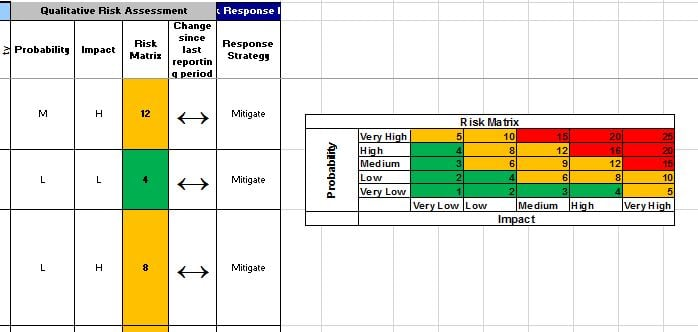or Explore Discussions

# Risk Assessment formula conversion from excel to smartsheet

03/21/19 Edited 12/09/19

I'm trying to convert an excel formula to use in smartsheet without much success.

The excel formula is:

=IFERROR(MATCH(\$F4,'Lookup Table'!\$B\$3:\$B\$7,0)*MATCH(\$G4,'Lookup Table'!\$B\$3:\$B\$7,0),"")

In short, I need the 'Risk Matrix' column in smartsheet to show the score where the probability and impact rating intersect.

I didn't create the excel file (or formula) and am not a formula expert as is probably obvious from my question.

Can anyone help?Tags:

• The easy way or the hard way?

the easy way is to switch from using text and use numbers. Instead of very low - very high it would be 1 - 5 and all you have to do is multiply them.

=[Column2]1 * [Column3]1

the hard way is to convert the text into numbers then multiply them

=if([Column2]1 = "Very High",5,if([Column2]1 = "High", 4,if([Column2]1 = "Medium", 3, if([Column2]1 = "Low",2,1)))) * if([Column3]1 = "Very High",5,if([Column3]1 = "High", 4,if([Column3]1 = "Medium", 3, if([Column3]1 = "Low",2,1

Neither of these requires your risk matrix. Just change the column names to what yours are, and change the text if you are using just the letter instead of the full text.

• If you really want to use the risk matrix for some reason, you need to use index match match.

=INDEX([Column4]1:[Column9]5, MATCH([Column2]1, [Column4]:[Column4], 0), MATCH([Column3]1, [Column4]5:[Column9]5, 0))

• I thought about doing it the easy way round but wanted to maintain the status quo. The second solution worked perfectly. Thanks so much for your help.

• Man, that is awesome! is there somehow you can share this smartsheet as a template?